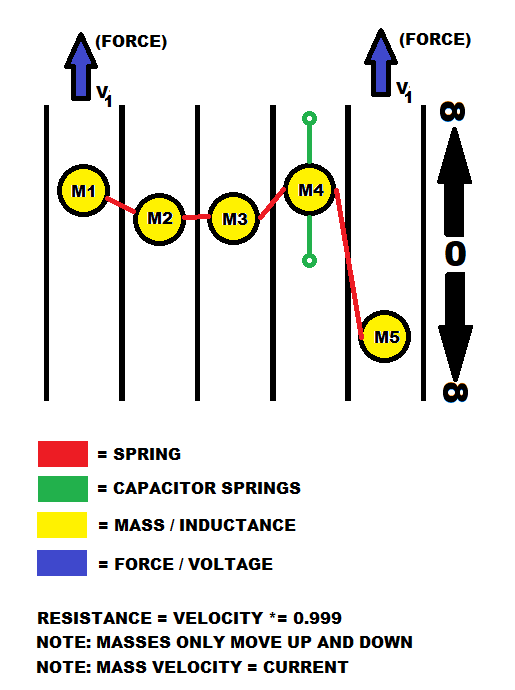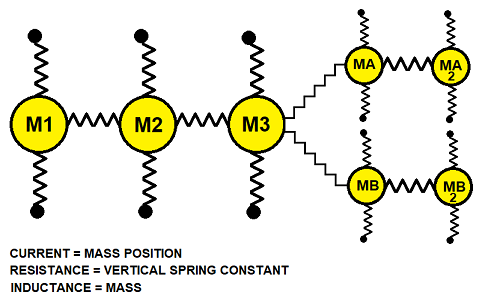# C:\> Rostislav Persion's Projects

.:: Spring Mass Model of Electricity ::.
A purely mechanical model of electricityI wanted to write a program in C# for simulating electrical circuits. I initially didn't know where to begin. I needed to make a scalable model which was both simple, yet all inclusive. After a whole day of thinking, I finally came up with a mechanical model that can perfectly mirror all electrical properties and was easy to simulate (Hooke's Law and 1 dimension particle simulation)... resistance, capacitance, inductance, diode component, and signal propagation.

Above is the diagram of the mechanical model.

Each mass has inertia just like an inductor. When you apply a voltage (force) to an inductor (mass) it takes time for the current (mass velocity) to build up through it. When you remove the voltage (force), the current (mass velocity) tries to continue flowing. The speed of each mass represents current in the finite element of wire. Each mass is connected to its neighbor by a spring. When a mass begins to move up, it starts to pull its neighbor up as well (with subtle latency). To simulate a capacitor, you need to anchor one of the masses with a spring. When you start pulling the anchored mass it begins to move but then slows down and stops. This is just like charging a capacitor using a DC power source. Current begins to flow freely but as the capacitor becomes charged the current comes to a stop eventually. Inductance (mass inertia) blocks AC. Capacitance (anchored mass) blocks DC, just like actual electronics. Resistance is the same as multiplying a mass velocity by a coefficient that has a value of less than one. You can also simulate a diode by only letting the masses move in one direction. Because of the latency between each mass interaction by means of spring, you can see signals propagate, just like in real life. To form a closed circuit, you must connect the left most mass to the right most mass.

Resistance: Mass velocity = mass velocity X Resistance Coefficient

Capacitance: Spring anchored mass

Inductance: Mass inertia

Diode: Let mass only move in one direction

Switch: Resistor of high value, when button contacts are open

Some issues with the model... If current is constant the mass location will go off into infinity and the computer program will crash due to the overflow. A solution for this is to make the motion circular instead of linear, how ever this adds more complexity.

I am also unable to figure out how to model parallel circuits. If you figure it out please let me know.

### MODEL 002Throttle method... This model works well in parallel. I cannot however find a way to simulate capacitance with this model.